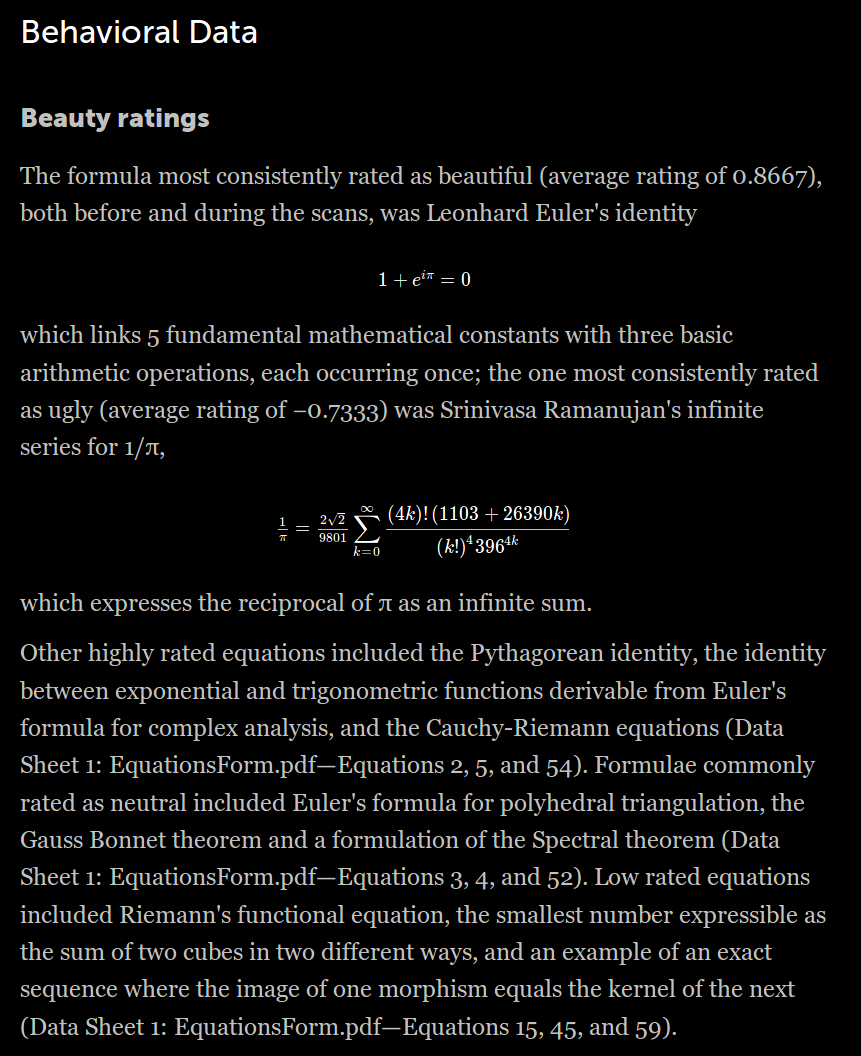M A T H 2 1 B
Mathematics Math21b Spring 2017
Linear Algebra and Differential Equations
Exhibit: Beauty in Mathematics
Office: SciCtr 432

# Beauty in MathematicsA NYT article from April 15, 2017 about a psychological study which showed that consistently, (using brain scans of mathematicians) that the most beautiful formula is
``` 1 + ei π = 0
```
(Scroll down to see one of the sections in that article featuring also a formula of Ramanujan on π). This formula is a special case of ei θ = cos(θ) + i sin(θ. We used the later to find the eigenvalues and eigenvectors of circular matrices. We did not tell you then but what you did there is use the discrete Fourier transform already.

By the way, the Fourier formulas are beautiful, but they become especially appealing if one goes to the complex. Instead of the basis
```{ cos(n x), sin(nx), 2-(1/2) } ,
```
one can also use the basis
```   ei n x
```
A function f(x) has then the Fourier expansion
```   f(x) = ∑n cn  ei n x
cn = (2 π)-1 ∫-ππ f(x) e-i n x dx
```
which is beautiful too. For some, Fourier theory is at first more like a beast but that changes once one sees through it.